## Tuesday, 29 May 2012

### Osu!: Taiko Mapping - drum breaks

Declaration: Taiko mapping is a place for creativity, existing no objective rules on good/bad map, and all to be discussed are individual opinion. Of course, comments are highly welcomed.

This is my first trial to write something on Taiko mapping --- in fact very difficult unless we take out a piece of music sheet can place each note corresponding to music, so I tried to take about some particular section instead.

What is a drum break? In a song full of instrumental (or vocal) sounds, we may found a section with no sound except a instrumental solo. It could be piano, drums, violin or whatever. That section can be stated as a transition, or a temporary period that takes auidences out of the original musical flow. And for the case of drums, it could be some stable 1/2 beats or a pure solo show-off.

The following youtube vid is from Go West, a section where Chico plays the piano with apple

Around 1:00 you can see Chico making some beats instead of purely piano performance, and that is an impressive transition in the song.

In a taiko mapping environment of course you can't switch the instrument freely (and I personally object custom hitsounds even they are unique throughout the song because taiko is a simulation of taiko -- a tradtional japanese instrument -- and is unique.) so what you can do is switching the reference of mapping -- from a melody based approach to a semi-solo approach.

Sometimes I emphasize how the taiko fit with the song (song comes first because you used that song and you are not creating the song under entire taiko diff), and taiko diff shouldn't cover the song. With a strong melody flow taiko sound act as a nice assistant to the song, and in weaker part you process more variation and stream to the next section and that provides a more interesting diff rather than a fixed result from a song.

In vocaloid songs ABABCDAB approach is common. Assuming B is the chorus and that means you are pushing the pace to the climax at the third kiai time. C is typically instrumental, D is a slower vocal so a solo style on section C contrast to D and gradually a denser and stronger beat to B is one of the approach to produce an well-organized climax at the beginning of B (and difference should be shown comparing with the first two kiai in the final section, and their pitch sometimes rised. Example: Demetori - innumerable eyes (though this is not vocaloid but the section dividing is similar http://osu.ppy.sh/s/21480).

For IIDX or BMS songs they have a stronger beat throughout the song (because they are made for BMS games so beats are needed everywhere) so you might not have the chance to produce such a constrast, and we will discuss them later if possible. (Well, these songs already give enough chance for mapper to tweek the drum pattern a bit and deliver a solo-styled diff) Sometimes people always complain that following the song strictly is boring, but I don't really think so. A suitable choice of reference would be enough to give a nice diff without some intentional drum solo.

## Saturday, 19 May 2012

### Basics of integration. Part III. Ordinary Substitution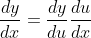Example 1. (Chain rule) Evaluate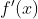where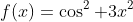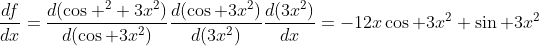Algebrically reading the equation it looks like "multiplying and divising the same thing to the entire function like rationalization and transforming complex number to standard form.

Apply chain rule to a composite function: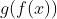. By chain rule we have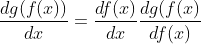If you are not sure about what the second term means, do a substitution u=f(x) and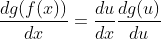.

Now integrate both sides once: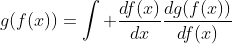(notice that RHS can cancel out each other to give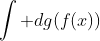which clearly gives the term at LHS.)

We can transform the above equation to another common representation for easier reading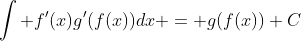.

Theorem 1. Integration by substitution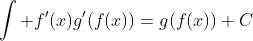.

## Tuesday, 15 May 2012

### M2: Algebra and geometry Part 2

When the rate of change considering the segment length is considered, one of the direct application is mechanical deduction. Differentiation build up a strong relationship between displacement, velocity and acceleration so differential equation would be useful here.

Locus of a certain point in coordinate geometry is a big topic in core/M2 mathematics, and it has significant applications, remarkably some pivot systems.

Example 3a. (Locus on linear system) Let A, B and C be 3 points on positive x-axis, positive x-axis and negative x-axis respectively. AB = 5, BC = 7. Let |OA| = x, |OC| = y, find dy/dx.

What I want to illustrate here is that, the differentiation operator has a very nice behavior that allows us to do questions in many ways. Denote |OB| = h.

Solution 1. Set up relation between x,y through h.
Obviously there are pairs of right angled triangle, hence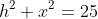and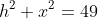, then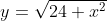(since y is positive), then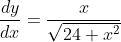.

Solution 2. Implicit differentiation.
First we set up the explicit relation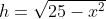and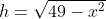. By differentiatingw.r.t. t,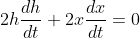, and do the same thing to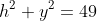to get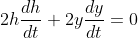.

Now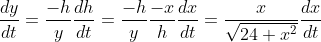Therefore. Note that we can multiply both sides by dt/dx, and eliminate the terms dt by chain rule.

Solution 3. Differentation through angle.
Let angle BAC be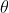. Then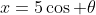,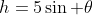,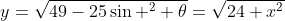. Now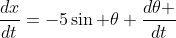,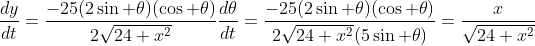.

The same question can be solved by different approaches, and that's the art of problem solving.

## Friday, 11 May 2012

### M2: Algebra and geometry Part 1

In HKCEE A-maths or HKDSE M2 paper, the topic "rate of change" as the application of differentiation always appear in the paper and sometimes it has been modelled into a geometric question like the velocity of a certain position of a pivot. What I want to illustrate is that geometric question can be modelled into a algebra question in many ways like vectors, coordinate geometry, and this time we can deal with calculus.

With the assistance of trigonometry we built up a strong relationship between segment lengths and angles, allowing us to relate different quantity, then the rate of change of a certain quantity can be transformed easily. We will demonstrate four questions, one unrelated to calculus, one A-math, one M2 and one Tokyo U questions, showing that differentiation can deal with meaningful conclusion instead of simply finding some derivatives.

Recall: Sine law and Cosine law.

Theorem. (Sine Law)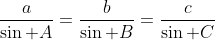Theorem. (Cosine Law)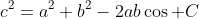Question 1. (Incircle) Let a,b,c be three sides of right-angled triangle ABC where c is the hyp. side. Show the incircle radius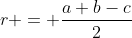Define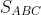be the area of the triangle ABC.

Now notice that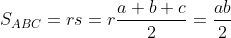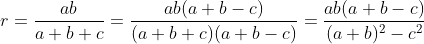Since it is right angled triangle,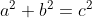, and therefore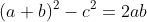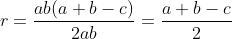Comparing with the x-y-z approach of incircle (Fact: AF = AG, CF = CE, BG = BE due to tangent properties or congruent triangle.) this is completed in a more algebrical way. (Using the x-y-z approach requires a cartesian plane which the right angled properties is shown in a geometrical simulation, but in this approach it is shown in a algebrical way).